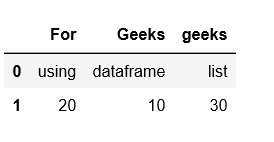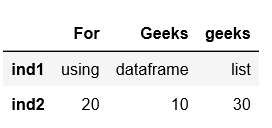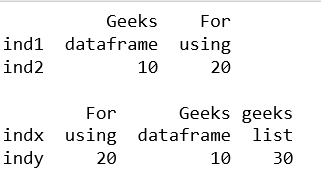# Create a Pandas DataFrame from List of Dicts

Pandas DataFrame is a 2-dimensional labeled data structure with columns of potentially different types. It is generally the most commonly used pandas object.

Pandas DataFrame can be created in multiple ways. Let’s discuss how to create a Pandas DataFrame from List of Dicts.

Code #1:

 `# Python code demonstrate how to create   ` `# Pandas DataFrame by lists of dicts.  ` `import` `pandas as pd  ` `   `  `# Initialise data to lists.  ` `data ``=` `[{``'Geeks'``: ``'dataframe'``, ``'For'``: ``'using'``, ``'geeks'``: ``'list'``}, ` `        ``{``'Geeks'``:``10``, ``'For'``: ``20``, ``'geeks'``: ``30``}]  ` `   `  `# Creates DataFrame.  ` `df ``=` `pd.DataFrame(data)  ` `   `  `# Print the data  ` `df  `

Output:Code #2: With `index`

 `# Python code demonstrate how to create   ` `# Pandas DataFrame by lists of dicts.  ` `import` `pandas as pd  ` `   `  `# Initialise data to lists.  ` `data ``=` `[{``'Geeks'``: ``'dataframe'``, ``'For'``: ``'using'``, ``'geeks'``: ``'list'``}, ` `        ``{``'Geeks'``:``10``, ``'For'``: ``20``, ``'geeks'``: ``30``}]  ` `   `  `# Creates DataFrame.  ` `df ``=` `pd.DataFrame(data, index ``=``[``'ind1'``, ``'ind2'``])  ` `   `  `# Print the data  ` `df  `

Output:Code #3: With `index` and `columns`

 `# Python code demonstrate how to create   ` `# Pandas DataFrame by lists of dicts.  ` `import` `pandas as pd  ` `   `  `# Initialise data to lists.  ` `data ``=` `[{``'Geeks'``: ``'dataframe'``, ``'For'``: ``'using'``, ``'geeks'``: ``'list'``}, ` `        ``{``'Geeks'``:``10``, ``'For'``: ``20``, ``'geeks'``: ``30``}]  ` `   `  `# With two column indices, values same   ` `# as dictionary keys  ` `df1 ``=` `pd.DataFrame(data, index ``=``[``'ind1'``, ``'ind2'``], ` `                      ``columns ``=``[``'Geeks'``, ``'For'``])  ` `    `  `# With two column indices with   ` `# one index with other name  ` `df2 ``=` `pd.DataFrame(data, index ``=``[``'indx'``, ``'indy'``])  ` `    `  `# print for first data frame  ` `print` `(df1, ``"\n"``)  ` `    `  `# Print for second DataFrame.  ` `print` `(df2)  `

Output:My Personal Notes arrow_drop_upCheck out this Author's contributed articles.

If you like GeeksforGeeks and would like to contribute, you can also write an article using contribute.geeksforgeeks.org or mail your article to contribute@geeksforgeeks.org. See your article appearing on the GeeksforGeeks main page and help other Geeks.

Please Improve this article if you find anything incorrect by clicking on the "Improve Article" button below.

Article Tags :

Be the First to upvote.

Please write to us at contribute@geeksforgeeks.org to report any issue with the above content.